# How to Activate Relay using Transistor?

As I searched the internet, transistors were configured in common-emitter configuration. They also show me that in order to activate the relay, the transistor should be operated in saturation and cut-off mode. How to make my BC547 Transistor operate to saturation mode (using common-emitter configuration) in order to activate my 24 VDC Relay using 24 VDC Source? How to decide proper Resistor Values?

NascentOxygen
Staff Emeritus
Hi nicy12. Your research covers a lot of the details. One more detail you need to supply is the source of the switching signal. What will you be using to control the switching transistor? A microswitch? A logic signal from some circuit? How many volts?

Your relay opeartes off 24VDC, but how much current does its solenoid draw while in operation?

Not enough information provided yet.

BTW, you'll also need a protection diode, because solenoids generate a spike in the voltage across the winding when you switch the current off

•davenn
davenn
Gold Member
2021 Award
here's a couple of ideas I did for some one else
it will give you a starting point
BUT you need to answer NascentOxygen's questions so transistor type etc can be suggested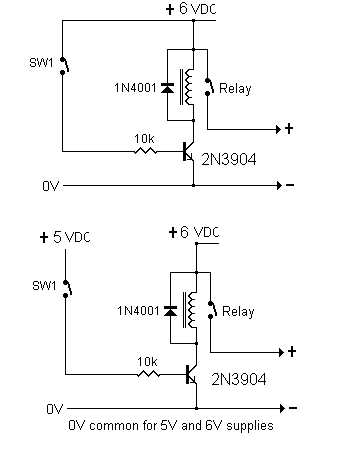cheers
Dave

Most of your project sized 24 V relays need about 60 ma, 150 ma on the outside. The transistor should be fairly tough. A 2N2222 has a 500ma limit, which is pretty tough.
The following is a good starting point and can be tweeked to fit your needs: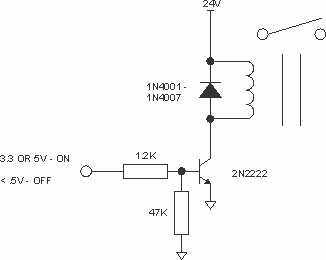Note that you'll need to supply at least 2 ma at 3.3V to be sure of driving a 150 ma coil.

Hi nicy12. Your research covers a lot of the details. One more detail you need to supply is the source of the switching signal. What will you be using to control the switching transistor? A microswitch? A logic signal from some circuit? How many volts?

Your relay opeartes off 24VDC, but how much current does its solenoid draw while in operation?

Not enough information provided yet.

BTW, you'll also need a protection diode, because solenoids generate a spike in the voltage across the winding when you switch the current off

Sir NascentOxygen I want my 24 VDC Source to be the source of relay as well as transistor. The circuit should use only one Voltage Source. Regarding Relay, I don't know what details in the data sheet I downloaded (my relay is MY4N-J) were needed to be used so I will post an image so you can help me about this: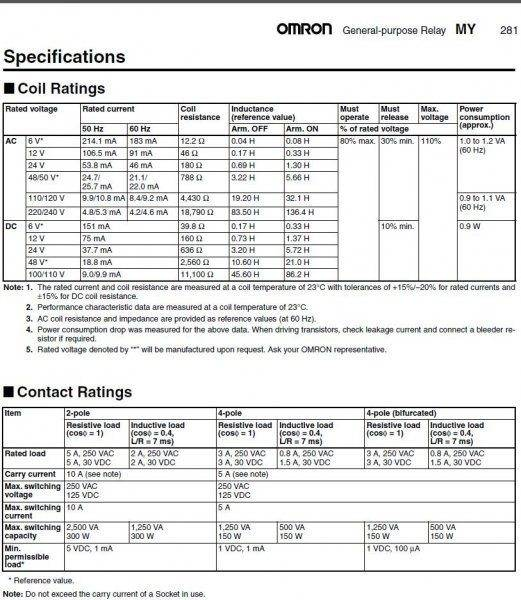The Relay I am going to used has LED inside the casing that serve as an indicator so I think it also serve as a flyback diode.

As I searched the internet, transistors were configured in common-emitter configuration. They also show me that in order to activate the relay, the transistor should be operated in saturation and cut-off mode. How to make my BC547 Transistor operate to saturation mode (using common-emitter configuration) in order to activate my 24 VDC Relay using 24 VDC Source? How to decide proper Resistor Values?

This is the circuit (which I designed) I really want to build: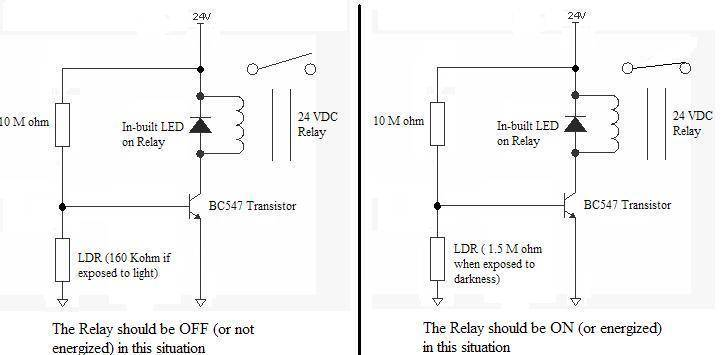As much as possible I don't want to replace the Transistor but if it was really needed so I will follow. The LDR values given was tested by me so it should be fixed.

NascentOxygen
Staff Emeritus
Yes, that 37.7 mA looks to be the current the relay coil needs.

But before going further, can you confirm that you already possess that LDR? They are not used so much now, I seem to recall them having limited long-term reliability. More common now are phototransistors.

If you have purchased that LDR, then you may require more than one transistor. You'll need to test the LDR for its dark and light resistances, under the light conditions you'll be relying on. Those R values in the schematic are probably just examples in a textbook figure.

Whatever the LED indicator does, you should not rely on it. Add the protection diode.

Last edited:
Yes, that 37.7 mA looks to be the current the relay coil needs.

But before going further, can you confirm that you already possess that LDR? They are not used so much now, I seem to recall them have limited long-term reliability. More common now are phototransistors.

If you have purchased that LDR, then you may require more than one transistor. You'll need to test the LDR for its dark and light resistances, under the light conditions you'll be relying on. Those R values in the schematic are probably just examples in a textbook figure.

Whatever the LED indicator does, you should not rely on it. Add the protection diode.

Yes I already bought one. The LDR values given were tested according to the situation or place where it will be placed. I am the one who tested it using multimeter ( I taped the LDR in the probes of my tester and measure its resistance at the location when there is light and when there is no light).

I used 10 M ohm Resistor because it will give me approx. 0.4 V at the base when there is light and approx. 3 V when there is no light ( greater than 0.7 of Vbe)

I have no idea on using phototransistor so research should be done in order to build circuit regarding that. I first want the LDR-Relay combination to this home project. I used transistor becuase my research says that transistors are also used in driving Relays.

If I am going to add 1N4001 Diode, Where it would be placed in the circuit?

here's a couple of ideas I did for some one else
it will give you a starting point
BUT you need to answer NascentOxygen's questions so transistor type etc can be suggested

View attachment 77885

cheers
Dave

Sir Davenn does your circuit really drive the 24 VDC relay? Because you are only using 6 VDC as Vcc. Please explain.

NascentOxygen
Staff Emeritus
I can't get your circuit to work with even a pair of BC547s. I think it can be made to work by substituting a JFET for the BC547. The FET won't load down your divider network.

I don't have any favourite FETs for this voltage, but the 2N7000 seems available and should do the job.

http://www.futurlec.com/Transistors/2N7000.shtml

Are you in a position to purchase that FET?

I am afraid to use FET because I haven't understand the BJT cleary in terms of practice. I have a little idea about BJT's in terms of theory where the type of transistor don't matter because needed parameters are given to solve the problem. To be specific, I don't know what details in the data sheet should be considered thus, I don't know what type of transistor should be used. I just found that BC547 as part of Dark Sensor Circuit here:
http://electronicpowersupply.blogspot.com/2014/04/simple-light-dark-detector-circuit.html
So I searched for the data sheet of that transistor here:
http://www.futurlec.com/Transistors/BC547.shtml
I found out that BC547 has maximum Emitter-Base Voltage of 6 V (I think that would be the maximum Vbe) and the minimum Base-Emiiter On Voltage was 0.55 V ( I believe that it was the needed Vbe to turn the transistor ON).

My problem now is I don't know what kind of transistor is going to be used so that I can drive the 24 VDC 3.7 mA Relay. Some website says that I should make my transistor to operate in Cut-off and Saturation Region. Operating in Cut-off is easy, I just need to Cut the supply in base (or supply it with less than 0.7 V). But operating in Saturation Mode makes me feel dumber given that I have already resistor values in the circuit.

I also don't want to use FET because I don't have any idea about it except it switches faster than BJT. Well, I don't want to mess in FET while I am having trouble to BJT.

One more thing, I honestly don't get it:
"I can't get your circuit to work with even a pair of BC547s. I think it can be made to work by substituting a JFET for the BC547. The FET won't load down your divider network."

Svein
This is the circuit (which I designed) I really want to build:

View attachment 77901

As much as possible I don't want to replace the Transistor but if it was really needed so I will follow. The LDR values given was tested by me so it should be fixed.

Just off the top of my head - that 10M resistor seems way too large. Try removing the LDR (simulating "extremely dark") and see if the circuit energizes. I suspect it will not. The trouble lies in the current amplification of the BC 547. 24V through 10Mohm results in a current of 2.4μA. If the β of BC 547 is 200, then the collector current will be 4.8mA - this not nearly enough to energize the relay (the spec sheet says 37.7mA at 24V).

NascentOxygen
Staff Emeritus
I used 10 M ohm Resistor because it will give me approx. 0.4 V at the base when there is light and approx. 3 V when there is no light ( greater than 0.7 of Vbe)
It's all very well to look at the two resistor divider alone, but when you connect it to the transistor base that transistor must draw some current from the divider, and this messes up your calculations. The base needs a lot more current than your network can provide, so the resistor values are no good, and then the light vs. darkness voltage variations won't be what you'd hoped. Alas, it just won't work.

A FET is simpler, it doesn't need much gate current at all, so it will work just like you'd hoped!

Just off the top of my head - that 10M resistor seems way too large. Try removing the LDR (simulating "extremely dark") and see if the circuit energizes. I suspect it will not. The trouble lies in the current amplification of the BC 547. 24V through 10Mohm results in a current of 2.4μA. If the β of BC 547 is 200, then the collector current will be 4.8mA - this not nearly enough to energize the relay (the spec sheet says 37.7mA at 24V).

Why should I removed the LDR if I am going to simulate an "exteremely dark" situation if I can substitute it to be 3 M ohm (as I tested it in very very dark location)?
$$\frac{3x10^6 x 24}{10 x 10^6 + 3 x 10^6} = 5.54 V$$
It is enough to turn the transistor on without burning it (since the maximum is 6 Vdc)

Svein
3x106x2410x106+3x106=5.54V
\frac{3x10^6 x 24}{10 x 10^6 + 3 x 10^6} = 5.54 V

That is correct - if you look at the resistor string alone. But the transistor base will lock at 0.7V and from there it is a question of supplying enough base current to the transistor. In order to turn on the relay, the transistor has to supply 38mA. Assuming a conservative β of 100, you need to supply 0.38mA into the base. 24V/0.38mA equals 63kΩ. 10MΩ is therefore way too large.

You could use an extra BC 547 as a source follower in front of your existing BC 547. Again assuming a β of 100, you need to supply 3.8μA into that base - which lets you get away with 6.3MΩ. Still less than 10MΩ, and you are now using a base current of the order of the leakage currents.

A FET is simpler, it doesn't need much gate current at all, so it will work just like you'd hoped!

A FET draws no gate current at all, but it has other problems. A JFET needs to operate with a negative gate voltage and it has no defined "turn-on" point. An "enhancement-mode" MOSFET will work with a positive gate voltage, but it still has no clearly defined "turn-on" point.

Personally, I would have used a comparator and fed the comparator output into the BC 547 base.

My little brain will going to burn! I can't make any circuit that comply the requirements.. If I will consider the voltage in Base-Emiiter Junction, there will be no enough current. If I'll consider the current, the voltage in Base-Emitter Junction is high that will burn the transistor. What should I do?

Personally, I would have used a comparator and fed the comparator output into the BC 547 base.

Can you provide me the circuit Sir?

You could use an extra BC 547 as a source follower in front of your existing BC 547. Again assuming a β of 100, you need to supply 3.8μA into that base - which lets you get away with 6.3MΩ. Still less than 10MΩ, and you are now using a base current of the order of the leakage currents.

I tried to use the 6.3 M ohm you said and this is the circuit I produced. Please tell me if I made it correctly: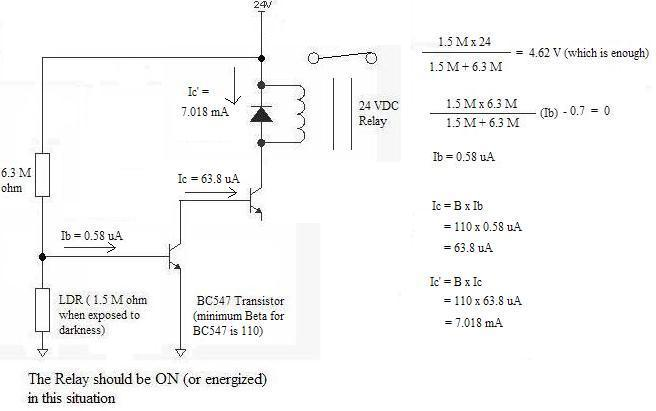7.018 mA is also not enough to activate my Relay. If I will going to add another, this will activate the relay but it is not convenient because three (3) transistors were used.

Last edited:
Svein
Sorry. The first transistor has to be connected as an emitter-follower, which means: Collector to +24V, base as you have drawn it and emitter connected to the base of the other transistor (with a 10kΩ resistor to ground).

Sorry. The first transistor has to be connected as an emitter-follower, which means: Collector to +24V, base as you have drawn it and emitter connected to the base of the other transistor (with a 10kΩ resistor to ground).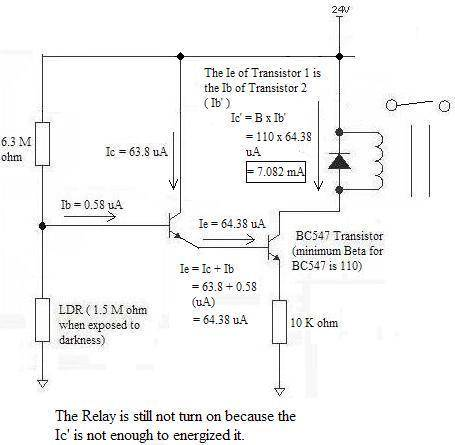Is it correct now? If not please tell me so I can do it again so that the circuit will be working.

Svein
View attachment 77918

Is it correct now? If not please tell me so I can do it again so that the circuit will be working.
The 10k should go from the emitter of the first transistor to ground (the only reason for it is to remove leakage current).

On another note: your calculations are wrong. The base of the first transistor never goes more that 1.4V above ground. This means that a 6.2M (6.3 is not a standard value) resistor will source 3.7μA. This means that you get 370μA out on the first emitter and therefore 37mA into the collector of the second transistor.

I have installed a small schematic drawing program and drawn a simple circuit using a comparator.

#### Attachments

• comparator.pdf
102.1 KB · Views: 210
Averagesupernova
Gold Member
A single transistor should do it. You need to get the 10M / 6.3M resistor small enough so that it can source all the current needed into the base of the transistor along with some through the dark LDR all the while having enough base current for the lowest case beta transistor.
-
Assume you will have a minimum beta of 50. The relay coil needs 38 mA. This means that the base needs .76 mA to activate the relay. A grounded emitter resistor will never have the base higher than about .7 volts. 24 volts - .7 volts = 23.3 volts across the 10 M resistor. The current is much much less than the needed. Shrink the resistor to about 27K and the transistor will turn on. The 1.5 M LDR should not divert too much current away from the base in darkness and when lit should present a small enough resistance to keep the base voltage from getting high enough to turn the transistor on at all.

On another note: your calculations are wrong. The base of the first transistor never goes more that 1.4V above ground.

according to this website:
https://www.wisc-online.com/learn/c...entals--voltage-divider-biased-npn-transistor

I can found ##V_{BB}## (input voltage at the base via voltage divider resistors) using $$V_{BB} = \frac{R_2 * V_{CC}} {R_1 + R_2}$$

So I think it's possible to have voltage greater than 1.4 V at the base of the first transistor because $$\frac {1.5*10^6 \ *\ 24} {1.5*10^6\ +\ 6.2*10^6}\ =\ 4.68\ V$$
If I falsely understand the concept about the base of a transistor, please kindly tell me the right thing.

I don't get why putting 6.2 M ohm gives 3.7 uA? It means that you are dividing the 24 V directly to the 6 M ohm $$(\frac {24 V}{6.2x10^6} = 3.87 uA)$$ (it can be said that 3.87 uA is also equal to 3.7 uA) but the real thing is you get 3.7 uA without considering the 1.5 M ohm and 10 K ohm connected to the first transistor.

Remaking the circuit diagram while following what you said.. Is this the right circuit (as you said) ? :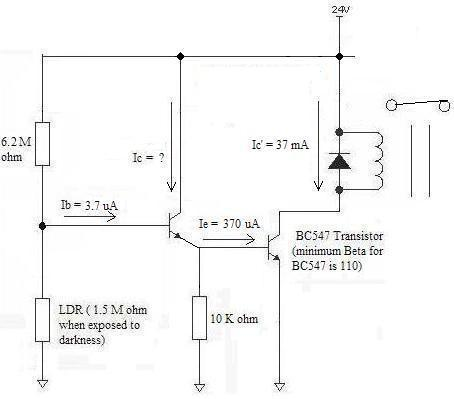If there is any problem please tell me. I am very determined to accomplish this circuit.

Averagesupernova
Gold Member
If you are not sure of collector current in the first transistor how can you know current going into the base of the second transistor?

If you are not sure of collector current in the first transistor how can you know current going into the base of the second transistor?

I just followed what Sir Svein describes.

A single transistor should do it. You need to get the 10M / 6.3M resistor small enough so that it can source all the current needed into the base of the transistor along with some through the dark LDR all the while having enough base current for the lowest case beta transistor.
-
Assume you will have a minimum beta of 50. The relay coil needs 38 mA. This means that the base needs .76 mA to activate the relay. A grounded emitter resistor will never have the base higher than about .7 volts. 24 volts - .7 volts = 23.3 volts across the 10 M resistor. The current is much much less than the needed. Shrink the resistor to about 27K and the transistor will turn on. The 1.5 M LDR should not divert too much current away from the base in darkness and when lit should present a small enough resistance to keep the base voltage from getting high enough to turn the transistor on at all.

If I am going to use 27 K ohm resistor, this will be the circuit :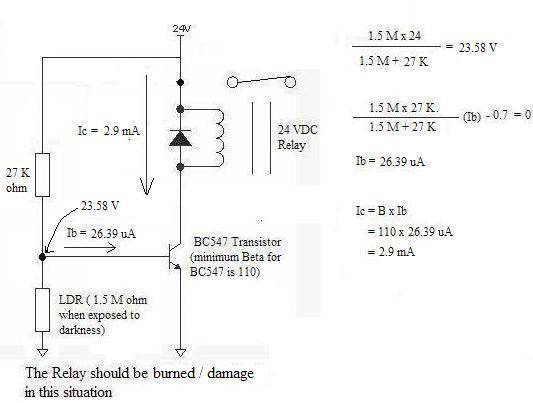The data sheet I posted earlier said that the voltage at the point will not to exceed 6 V. In the circuit above, it shows that the voltage at the point was 23.58 V.

The Beta (Current Gain) of BC547 is 110 (minimum) so the current in the collector will be 2.9 mA. It can't energized the relay which needs approx. 38 mA.

If I have errors in my calculations and If I have mistakes regarding the concept of BJT, Please kindly tell me. I really want to build this circuit.

Svein
according to this website:
https://www.wisc-online.com/learn/c...entals--voltage-divider-biased-npn-transistor

I can found ##V_{BB}## (input voltage at the base via voltage divider resistors) using $$V_{BB} = \frac{R_2 * V_{CC}} {R_1 + R_2}$$

So I think it's possible to have voltage greater than 1.4 V at the base of the first transistor because $$\frac {1.5*10^6 \ *\ 24} {1.5*10^6\ +\ 6.2*10^6}\ =\ 4.68\ V$$
If I falsely understand the concept about the base of a transistor, please kindly tell me the right thing.

I don't get why putting 6.2 M ohm gives 3.7 uA? It means that you are dividing the 24 V directly to the 6 M ohm $$(\frac {24 V}{6.2x10^6} = 3.87 uA)$$ (it can be said that 3.87 uA is also equal to 3.7 uA) but the real thing is you get 3.7 uA without considering the 1.5 M ohm and 10 K ohm connected to the first transistor.

Remaking the circuit diagram while following what you said.. Is this the right circuit (as you said) ? :

View attachment 77927

If there is any problem please tell me. I am very determined to accomplish this circuit.

The circuit you are linking to is very different. It talks about biasing a transistor to work as a linear amplifier. Your circuit is using the second transistor as a switch.

Basic info for a silicon transistor used as a switch: Vbe≈0.7V when the transistor conducts, Vbe≤0.2V when it does not.

Therefore, the maximum voltage at the base of the first transistor is Vbe (second transistor) + Vbe (first transistor) = 1.4V. Now you have one of your data points. The second data point: 38mA at the collector of the second transistor. This allows you to calculate the maximum value of the series resistance into the base of the first transistor.
Now I have cheated a little: I told you to remove the LDR in order to show that you would not be able to drive the relay with your first circuit. In an actual circuit, the value of the LDR in darkness (1.5MΩ) means that you drive the first transistor from a voltage of (1.5*24/7.7)V = 4.67V with a source impedance of (1.5M parallel with 6.2) = 1,2MΩ. This means that the current into the first base will be (4,67 - 1,4)V/1,2MΩ = 2,7μA. This means that we must reduce the 6.2M resistor still more - 4.7MΩ seems OK.

The next problem is to verify that the transistors will turn off in daylight. Using 4.7MΩ and your figure of 160kΩ, the voltage across an illuminated LDR would be 24V*(160/4860) = 0.8V which will very probably turn the circuit off.

Averagesupernova
Gold Member
In reviewing this thread I notice that the spec you put on the LDR is 160Kohm when exposed to light. Is this correct? Usually they are will below 1K when exposed to light. I made this assumption when I gave you the 27K value.

NascentOxygen
Staff Emeritus
In reviewing this thread I notice that the spec you put on the LDR is 160Kohm when exposed to light. Is this correct?
That is the figure OP has measured, so is what we're working with.

Usually they are will below 1K when exposed to light. I made this assumption when I gave you the 27K value.

If I willl going to use the 4.7 M ohm for the circuit, it runs very well if the LDR is exposed to darkness as it shows: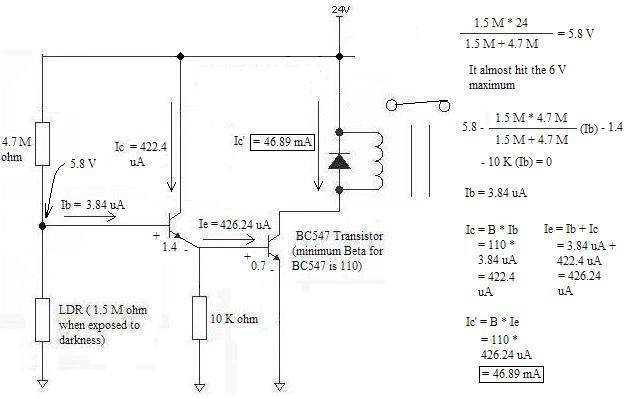The only problem here is the voltage in that point is 5.8 V, which is very close to the 6 V maximum rating as it shows here: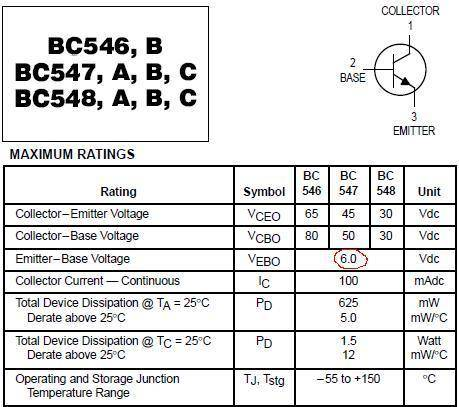The other problem in using 4.7 M ohm is when the LDR is exposed to light, the voltage develop in the point is enough to turn the transistor ON as well as it developed negative currents as it shows: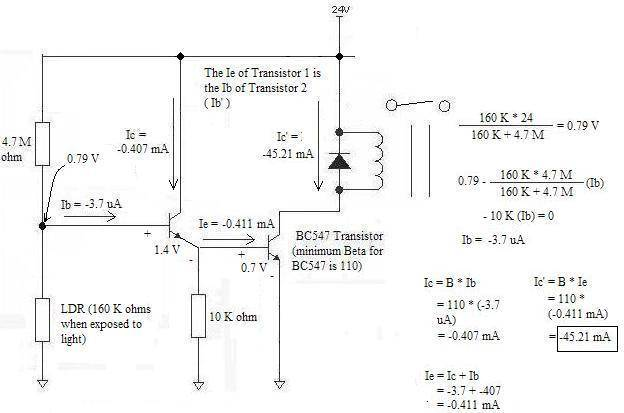the voltage across an illuminated LDR would be 24V*(160/4860) = 0.8V which will very probably turn the circuit off.

The voltage at that point should be less than 0.55 V as shown below: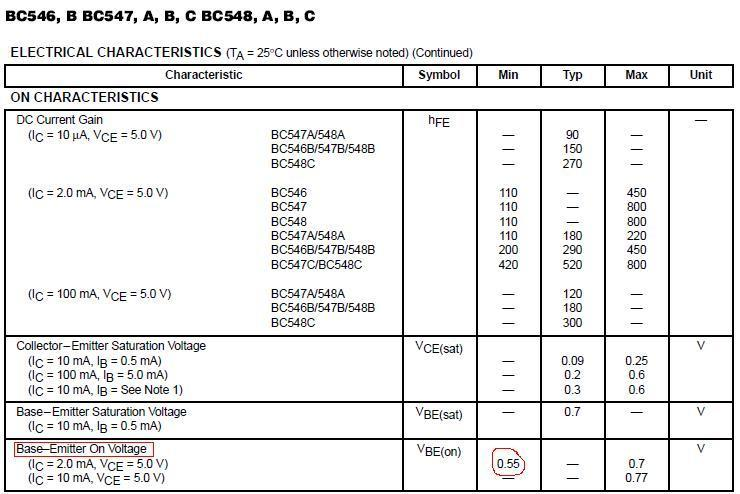If I have still errors in making the circuit as well as looking the right information in the posted data sheet, please inform me. I need to perfect this circuit for my own sake. Thank you.

Svein
If I have still errors in making the circuit as well as looking the right information in the posted data sheet, please inform me. I need to perfect this circuit for my own sake. Thank you.
Remember that you have two transistors here. Adding up two minimum values of Vbe gives 0.55V + 0.55V = 1.1V.

Remember that you have two transistors here. Adding up two minimum values of Vbe gives 0.55V + 0.55V = 1.1V.

So the 0.79 V in the exposed to light situation is not enough to turn on the transistor?

How about the negative values of currents? what is the explanation behind them?

Svein
The only problem here is the voltage in that point is 5.8 V, which is very close to the 6 V maximum rating as it shows here:

Sigh. No! The maximum voltage on the base of the first transistor is 1.54V (VBE(on) *2). Now, if you had connected the LDR to a negative voltage, you would have had to be careful of not getting below -6V...

Svein
So the 0.79 V in the exposed to light situation is not enough to turn on the transistor?

How about the negative values of currents? what is the explanation behind them?

• 0.79V should not be enough, no. If the transistor pair ends up conducting a little, reduce the 10k resistor.
• What negative currents?

Now, if you had connected the LDR to a negative voltage, you would have had to be careful of not getting below -6V...

Honestly, I really don't get the point. as the circuit above is concerned, the LDR is not connected to any negative voltage. The voltage on LDR is because of being in voltage divider configuration.

What negative currents?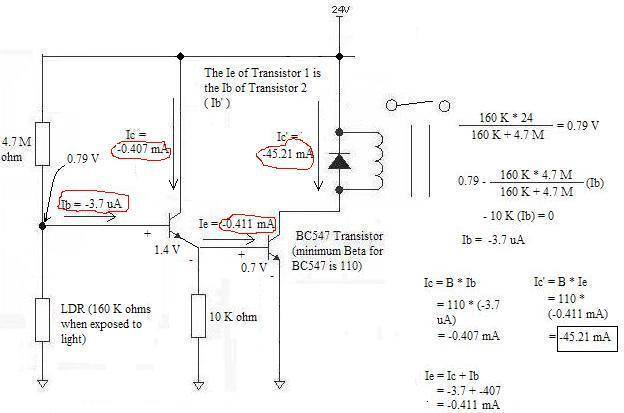Those in red is what "negative currents" I am talking about.